# Angle - Definition with Examples

The Complete K-5 Math Learning Program Built for Your Child

• 30 Million Kids

Loved by kids and parent worldwide

• 50,000 Schools

Trusted by teachers across schools

• Comprehensive Curriculum

Aligned to Common Core

## Angle Games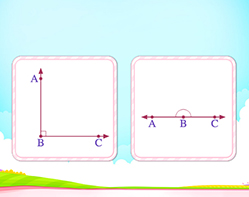Types of Angles

Classify angles on the basis of their measure, i.e. identify right angles, acute angles and obtuse angles.

Covers Common Core Curriculum 4.G.1

## What is an Angle?

In geometry, an angle can be defined as the figure formed by two rays meeting at a common end point.

An angle is represented by the symbol ∠. Here, the angle below is ∠AOB.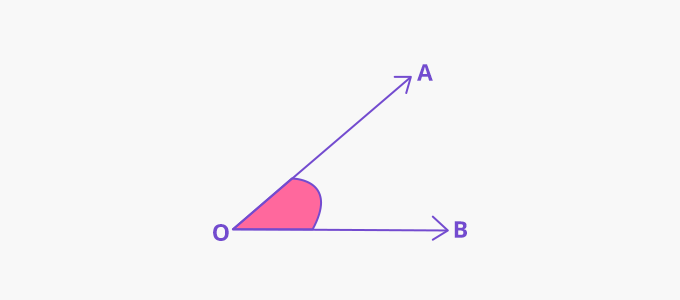Angles are measured in degrees, using a protractor.

## Parts of an Angle:

Arms: The two rays joining to form an angle are called arms of an angle. Here, OA and OB are the arms of the ∠AOB.

Vertex: The common end point at which the two rays meet to form an angle is called the vertex. Here, the point O is the vertex of ∠AOB.We can find angles in various things around us, such as in a pair of scissors, a hockey stick, a chair.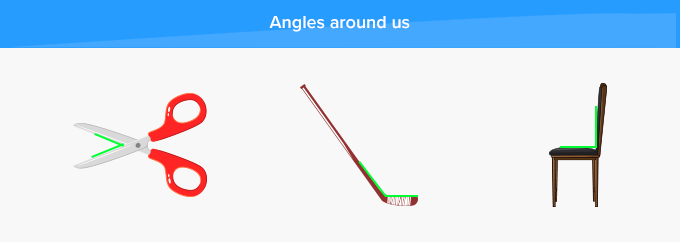## Types of Angles

Angles can be classified on the basis of their measurements as

- Acute Angles                               - Right Angles                            - Obtuse Angles

- Straight Angles                            - Reflex Angles                           - Complete Angles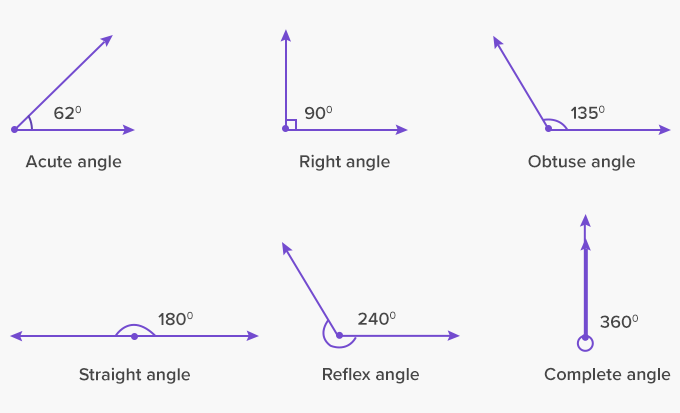## Interior and Exterior Angles:

Interior angles: Interior Angles are the angles formed within or inside a shape
Here, ∠ABC, ∠BCA and ∠CAB are interior angles.

Exterior angles: Exterior angles are the angles formed outside between any side of a shape, and a line extended from the adjoining side. Here, ∠ACD is an exterior angle.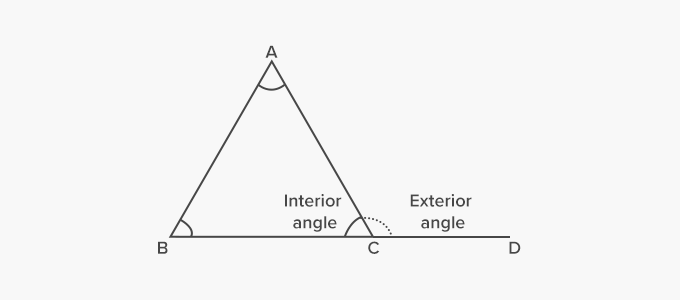Fun Facts The word ‘angle’ has been derived from the Latin word Angulus, meaning “a little bending”.  The concept of angle was first used by Eudemus, who defined an angle as a deviation from a straight line.

##Let's sing!

An acute angle is a little so small,
Right angle in the corner of a wall,
Obtuse angle at 2:50 in day-time,
Straight angle in a straw in soda-lime!
So many angles all around, even in a mime!

##Let's do it!

Instead of handing out geometry angles worksheets to your children, ask your child to observe/spot the things in which they can find different angles such as in a hanger, hands of a clock, or the roof a house.

##Related math vocabulary

Won Numerous Awards & Honors